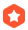# 连续邮资问题.间谍 长春市野狼科技有限公司 CTO  2001-08-03 07:29:12加精

...全文
397 点赞 收藏 13

13 条回复

starfish 2001-08-03

laozi 2001-08-03

starfish 2001-08-03

QBasic很吃亏的。我觉得DOS下面还是用turbo pascal 7.0 最好,TC3也不错，TC2就太差了：（

frman 2001-08-03
：））来蹭点参与分：

这个题就是搜啊搜啊，剪啊剪啊：）呵呵～～～～不过俺这方面不强，以前做的程序也不够快，这个题海星大哥发给过我他做的东西，虽然我还留着，不过就让海星大哥贴他的解析吧：）不过我看这好象刘汝佳没来（他喜欢ＩＤ就用自己名），而我也见过他站上写的这个东西的解析，贴出来看看吧：）

*****************************

max之间的每一个邮资值都能得到。

，所以MAX=7，面值分别为l分、3分。

INPUT
N=3 k=2
OUTPUT
1 3
MAX=7

P+1个面值也能得到S.

1.Cont(x)就是前x个元素的Q值
2.Find(Findstart,Contstart,x); x:当前搜索的邮票序号，findstart:第x张邮票的最小可能值（搜索起点），
Contstart:需要从Contstart开始连续取值（也就是：前x-1张可以从1取到Contstart-1）
OK，Here it is:

{\$A+,B-,D+,E+,F-,G-,I-,L+,N-,O-,R-,S-,V+,X-}
{\$M 65520,0,655360}

Program Stamp;
Const
Maxn=40;
Var
Value,BestValue : Array[1..Maxn] Of Integer;
Max,N,K : Integer;

{If N=5,K=5 Value[]=(1,4,9,31,51), It returns 126, Got it?}
Function Cont(x:Integer):Integer;
Var
I:Integer;
CanGet:Array[0..10000] Of Boolean;
Count:Array[1..Maxn] Of Integer;

Procedure UseIt(Num,Left:Integer);
Var
I:Integer;
Total:Integer;
Begin
If Num=x+1 Then
Begin
Total:=0;
For I:=1 to x Do Inc(Total,Value[i]*Count[I]);
CanGet[Total]:=True;
Exit;
End;
For I:=0 to Left Do
Begin
Count[Num]:=I;
UseIt(Num+1,Left-I)
End;
End;
Begin
Fillchar(CanGet,SizeOf(CanGet),0);
UseIt(1,n);
I:=1; While CanGet[i] Do Inc(I); Cont:=I-1;
End;

(*
This is the most important part
eg: N=3,K=3
Suppose we're searching for the 3rd stamp while the first two are 1,4
We got:
1 = 1
2 = 1+1
3 = 1+1+1
4 = 4
5 = 1+4
6 = 1+1+4
Then we call:

Find(5,7,3); that means, we should find a value for the 3rd stamp

from 5,6,7,8..., the first two stamps could get value 1..7-1.

Got it?
*)

Procedure Find(FindStart,ContStart,x:Integer);
Var
I:Integer;
ContMost:Integer;
Begin
If x=k+1 Then
Begin
If Max < ContStart-1 Then
Begin
Max := ContStart-1; {Hey, ContStart is NOT the largest number we really got!}
For I:=1 to K Do BestValue[I]:=Value[I]; {Copy to BestValue}
End;
Exit;
End;

I:=FindStart;
While True Do
Begin
Value[x]:=i;
ContMost:=Cont(x); {Calculate}
Find(I+1,ContMost+1,x+1);
If I+1>ContStart Then Exit Else Inc(I);
{if I+1>ContStart we CANNOT get value Constart 'cause I will be bigger and bigger}
End;
End;

Procedure Init;
Begin
Max:=0; {Initialize}
Value:=1; {The 1st Stamp Must Be 1 ^_^}
End;

Var I:Integer;
Begin
For I:=1 to K Do Write(BestValue[I],' ');
WriteLn;
WriteLn('MAX=',Max);
End;

Begin
Init;
Find(2,n+1,2); {Search From the 2nd Stamp}
End.

*************************

555555~~~~Pascal看不懂：）

frman 2001-08-03

****************************************

N K
7 3 1 8 13 MAX=69
7 4 1 5 24 37 MAX=165
10 3 1 10 28 MAX=146
5 5 1 4 9 31 51 MAX=126

***********************

starfish 2001-08-03

starfish 2001-08-03

#include <stdio.h>
#include <string.h>
#include <assert.h>

#define INPUT_FILE "stamp.in" // input file name
#define OUTPUT_FILE "stamp.out" // output file name
#define MAX_N 40

#define true 1
#define false 0

FILE *fin, *fout; // inout file and output file
int ProbN = 0; // the No. of input case
int n, k;

/*
stamp is current solution,
value[i][j] is the max value that using j pieces of stamp..stamp[i] will get
best is the best solution
*/
int stamp[MAX_N], value[MAX_N], best[MAX_N];

int MaxValue; // the max value of the best solution

{
if (feof(fin))
return false;

/*
*/
fscanf(fin, "%d%d", &n, &k);
if (n <= 0)
return false;

ProbN++;
return true;
}

int CanGet(int depth, int Y, int total)
{
if (depth == 0)
return (Y <= total);

for (int t=0; (t <= Y/stamp[depth]) && (t <= total); t++)
if ( CanGet(depth-1, Y-t*stamp[depth], total-t))
return true;
return false;
}

void CalValue(int depth)
{
value[depth] = value[depth-1];
while (CanGet(depth, value[depth]+1, n))
value[depth]++;
}

void search(int depth)
{
if (depth == k) {
if (value[k-1] > MaxValue ) { // record the best solution
memcpy(best, stamp, k*sizeof(int));
MaxValue = value[k-1];
}
return;
}

stamp[depth] = stamp[depth-1] + 1;
while (stamp[depth] <= value[depth-1]+1)
{
CalValue(depth);
search(depth+1);
stamp[depth]++;
}

}

void SolveCase()
{
MaxValue = 0;
stamp = 1;
value = n;
search(1);
fprintf(fout, "Case #%d\n", ProbN);
for (int i=0; i<k; i++)
fprintf(fout, "%d ", best[i]);
fprintf(fout, "max = %d\n", MaxValue);
}

int main()
{
assert( fin = fopen( INPUT_FILE, "r" ) );
assert( fout = fopen( OUTPUT_FILE, "w" ) );

SolveCase();

fclose(fin);
fclose(fout);
return 0;
}

NP问题！

Zig 2001-08-03

3.2w+